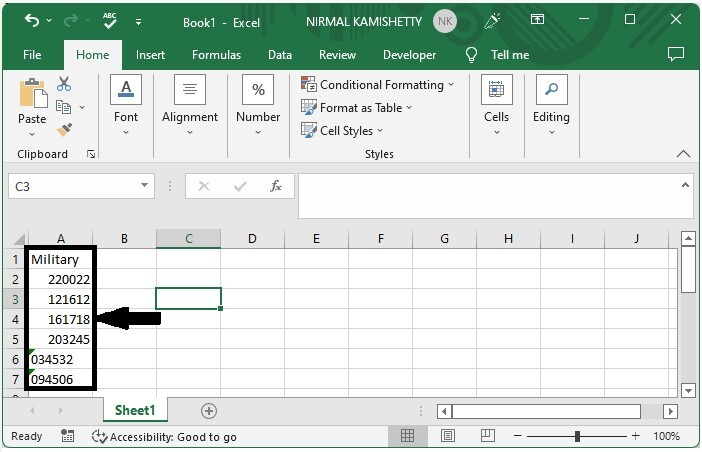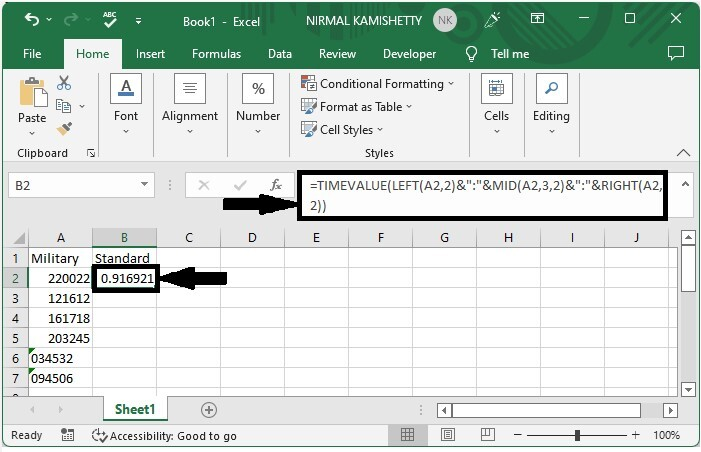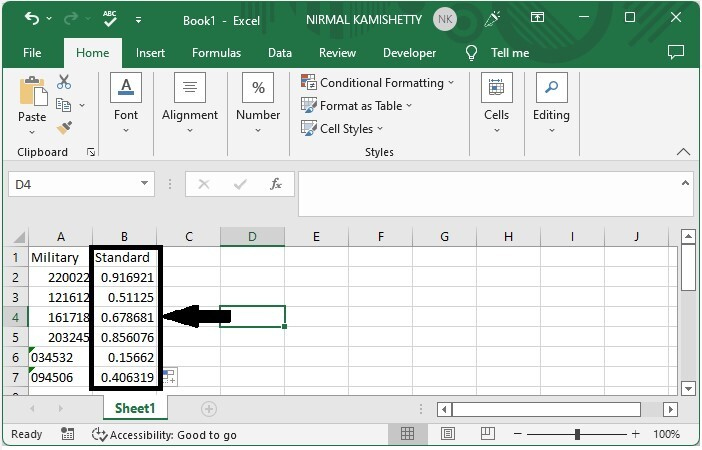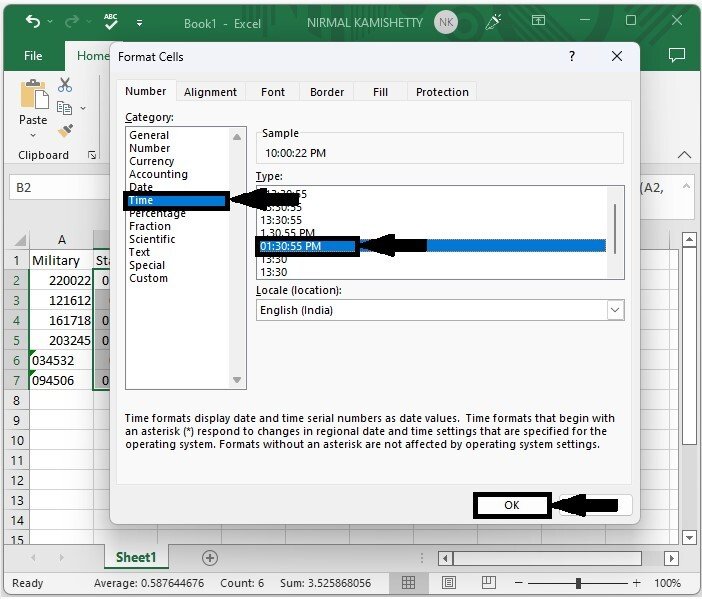# How to Convert Military Time to Standard Time in Excel?

If the time is represented in 24-hour format without any separator, then it can be called military time. If the times are in military format, then it is impossible to perform operations on them, and it can be confusing while we are understanding our data in the sheet. So, we need to convert them to a standard format for a more accurate understanding of the data. Read this tutorial to learn how you can convert military time to a standard format in Excel.

## Converting Military Time to Standard Time in Excel

Here we will use the TIMEVALUE formula to get the results, then use the format to convert them to time format. Let's look at a simple procedure for converting military time to standard time in Excel.

### Step 1

Let us consider an excel sheet where the data is a list of times in military format, similar to the below image.Now click on an empty cell, in our case cell B2, and enter the formula as =TIMEVALUE(LEFT(A2,2)&":"&MID(A2,3,2)&":"&RIGHT(A2,2)) and click enter to get our first result, which looks similar to the below image.

Empty cell > Formula > Enter### Step 2

Then drag down from the first result, using the auto-fill handle to fill all the values as shown in the below image.As we can see, the values are in number format, so we need to convert to time format.

### Step 3

To do so, select the values, then right-click and select format cells, then click on time and select the standard format from the list, and click OK to complete our process.

Select data > Right click > Time > Standard format > Ok### Note

If the given time has only hours and minutes, we can use the formula as

=TIMEVALUE(LEFT(A1,2)&":"&RIGHT(A1,2))


If we need to convert the given standard time to military time, we use the formula as

=TEXT(A1,"HHMMSS")


## Conclusion

In this tutorial, we used a simple example to demonstrate how you can convert military time to standard format in Excel.

Updated on: 06-Mar-2023

2K+ Views